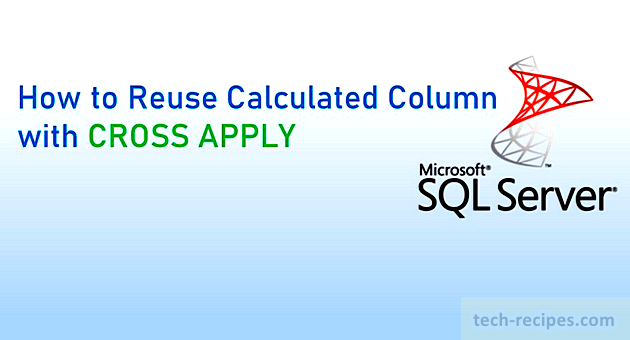# How to Reuse Calculated Column with CROSS APPLY In SQL Server

Posted November 3, 2020 by Vishwanath Dalvi in Database, SQL ServerAPPLY operators (CROSS APPLY & OUTER APPLY) were introduced in SQL Server 2005 edition. Importantly, with APPLY operators, now it is easier to join Table-valued function to base tables based on the join condition. Although APPLY operators different from SQL JOIN. In the case of SQL JOIN, we can not join to Table-valued function. CROSS APPLY operator is also used to reuse calculated column.

To enumerate the use of CROSS APPLY & OUTER APPLY, it calls Table-valued function for each row of a table in a join condition. To explain the following query, we can see that each row from sys.dm_exec_cached_plans view called by table-valued function sys.dm_exec_query_plan. Implementing this functionality using a regular JOIN is a little bit complex.

```--Using CROSS APPLY to join Tabled-Valued Function

SELECT plan_handle, query_plan, objtype
FROM sys.dm_exec_cached_plans
CROSS APPLY sys.dm_exec_query_plan(plan_handle)
GO
```

Provided the use of Table-valued function, we can utilize APPLY operators to simplify complex queries and write elegant code.

Numerous other use cases of APPLY operators are worth learning as following.

1. Unpivot the table data
2. Reuse column alias & calculated columns
3. Retrieving Top N records per group
4. Calling Table Valued Function for each row of the outer table

In this tech-recipe post, we are demonstrating how to reuse calculated columns with the CROSS APPLY operator.

1.

### Number Calculation – Reuse Calculated Columns CROSS APPLY

Further query demonstrates the use of CROSS APPLY to reuse calculated columns without actually repeating the code as we do in the regular query. We can reuse column alias because column alias evaluation happens inside an inner query with CROSS APPLY. Ideally, we can not reference a column name in SELECT directly considering the Logical Query Processing Order in SQL Server.

```--Normal Query - Repeated Column logic for Calculation

SELECT DISTINCT number, number + 1 as IncrementByOne, (number + 1) + 1 as IncrementByTwo
FROM   master..spt_values
WHERE number >=1 and number<= 10;

--Reuse calculated columns using CROSS APPLY

SELECT  DISTINCT number , CAP1.Y as IncrementByOne, CAP2.Z as IncrementByTwo
FROM    master..spt_values
CROSS APPLY (SELECT number + 1 as AddOne) CAP1(Y) --Reuse number column
CROSS APPLY (SELECT Y + 1 as AddOne)      CAP2(Z) --Reuse Cap1(Y) column
WHERE number >=1 and number<= 10;
```

2.

### SELECT Column Reuse – Reuse Calculated Columns CROSS APPLY

Here Query 2.1 tries to reuse the column name in the select list from left expression to right. However, it is not possible because of Logical Order Processing in SQL Server. Therefore, Query 2.1 is semantically incorrect and result in an error stating an invalid column name.

Reuse of column is possible with CROSS APPLY operator. In Query 2.2, reused the Number column without repeating the expression and so on.

```--Query 2.1 (Incorrect reuse of Column expression in SELECT)

SELECT 50 as Number, Number * 3 as NumberInto3, NumberInto3 * 5 as NumberInto3Into5;

--Query 2.2 (Works with column reuse CROSS APPLY)

SELECT TOP (1)
CA1.Number
,CA2.NumberInto3
,CA3.NumberInto3Into5
from  sys.tables
CROSS APPLY (SELECT 50) as CA1 (Number)
CROSS APPLY (SELECT (Number * 3)) as CA2 (NumberInto3)
CROSS APPLY (SELECT (NumberInto3 * 5)) as CA3(NumberInto3Into5);
```

3.

### Date manipulation – Reuse Calculated Columns CROSS APPLY

In the following query, retrieving a list of tables created between the 1st and 10th day of the current month. In Query 3.1– expression repeated twice to filter the records in where clause as well as in the select list. This increases code complexity and makes it less readable. We can avoid repeating code by simply using CROSS APPLY. Using the APPLY operator & reusing the calculated columns in where clause and select list in Query 3.2.

```--Find list of tables created between 1st and 10th day of current month

--Query 3.1 - (Repeat of expressions)

--Find list of tables created between 1st and 10th day of current month
SELECT name, CAST(create_date as DATE) as TableCreationDate, DATEADD(DAY,1,EOMONTH(getdate(),-1)) as FirstDayOfCurrentMonth, DATEADD(DAY,10,EOMONTH(getdate(),-1)) as TenthDayOfCurrentMonth
FROM sys.tables

--Query 3.2 - (Reuse of expersession)

SELECT name, CAST(create_date as DATE) as TableCreationDate, CA.FirstDayOfCurrentMonth, CA.TenthDayOfCurrentMonth
FROM sys.tables
CROSS APPLY ( SELECT DATEADD(DAY,1,EOMONTH(getdate(),-1)) as FirstDayOfCurrentMonth
,DATEADD(DAY,10,EOMONTH(getdate(),-1)) as TenthDayOfCurrentMonth ) as CA
where create_date >= CA.FirstDayOfCurrentMonth AND create_date <= CA.TenthDayOfCurrentMonth ;
```

4.

### CASE Statement – Reuse Calculated Columns CROSS APPLY

In this example, rewriting the same case statement expression 3 times to calculation bonus in Query 4.1. This increases complexity, and code becomes less precise. In Query 4.2, we have moved the CASE expression to CROSS APPLY and reused the set expression in the SELECT list to perform the further calculation.

```USE ContosoRetailDW;

--Repeated Case Expression
select Firstname
,BaseRate
,Title
,DepartmentName
,CASE WHEN Title = 'Sales Region Manager' AND DepartmentName = 'Marketing' THEN BaseRate * 2 ELSE 0  END AS Jan2021Bonus
,CASE WHEN Title = 'Sales Region Manager' AND DepartmentName = 'Marketing' THEN BaseRate * 3 ELSE 0  END AS Feb2021Bonus
,CASE WHEN Title = 'Sales Region Manager' AND DepartmentName = 'Marketing' THEN BaseRate * 4 ELSE 0 END  AS March2021Bonus
from ContosoRetailDW.dbo.DimEmployee;

--Avoiding repeat CASE expression using CROSS APPLY
SELECT Firstname
,BaseRate
,Title
,DepartmentName
,CA1.BenefitsCritria * 2 as Jan2021Bonus
,CA1.BenefitsCritria * 3 as Feb2021Bonus
,CA1.BenefitsCritria * 4 as March2021Bonus
from ContosoRetailDW.dbo.DimEmployee
CROSS APPLY (SELECT CASE WHEN Title = 'Sales Region Manager' AND DepartmentName = 'Marketing' THEN BaseRate ELSE 0 END) as CA1(BenefitsCritria)
```

### Summary

In a nutshell, we have learned how to use CROSS APPLY operator to reuse calculated columns. This makes code more easier to understand and avoids repeating of same logic. Moreoever, CROSS APPLY can do Unpivot and TOP N per group logic which will be covered in series of post. If you like this post you may browse through Tech-Recipes SQL Server Database archive to enhance your knowledge.